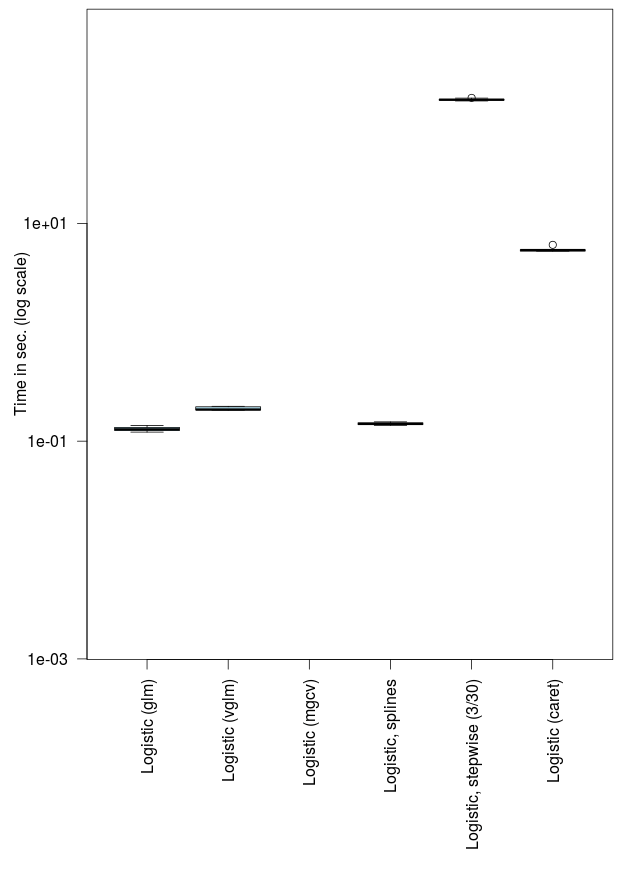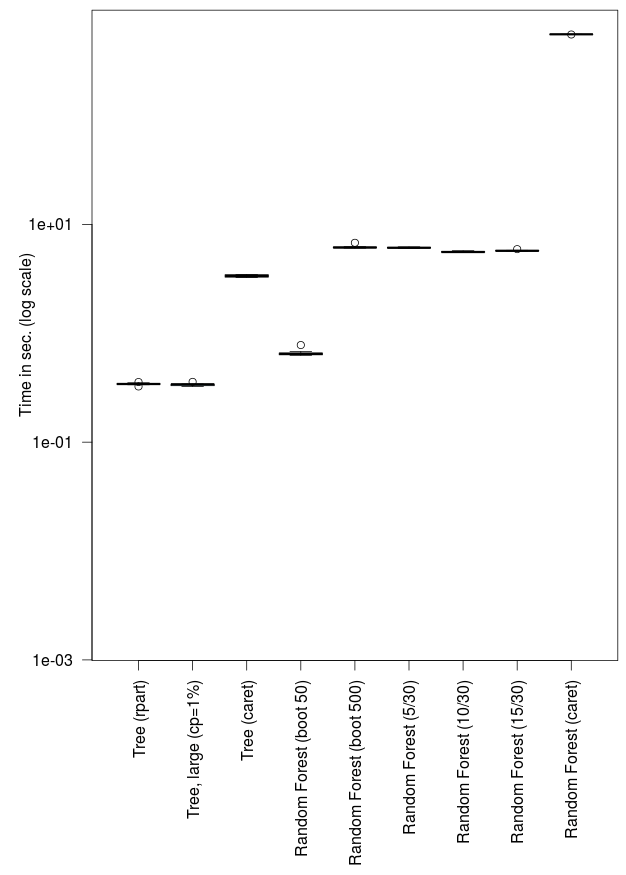[This article was first published on Freakonometrics » R-english, and kindly contributed to R-bloggers]. (You can report issue about the content on this page here)
Want to share your content on R-bloggers? click here if you have a blog, or here if you don't.

Tuesday, at the end of my 5-hour crash course on machine learning for actuaries, Pierre asked me an interesting question about computational time of different techniques. I’ve been presenting the philosophy of various algorithm, but I forgot to mention computational time. I wanted to try several classification algorithms on the dataset used to illustrate the techniques

```> rm(list=ls())
"http://freakonometrics.free.fr/myocarde.csv",
> levels(myocarde\$PRONO)=c("Death","Survival")```

But the dataset is rather small, with 71 observations and 7 explanatory variables. So I decided to replicate the observations, and to add some covariates,

```> levels(myocarde\$PRONO)=c("Death","Survival")
> idx=rep(1:nrow(myocarde),each=100)
> TPS=matrix(NA,30,10)
> myocarde_large=myocarde[idx,]
> k=23
> M=data.frame(matrix(rnorm(k*
+ nrow(myocarde_large)),nrow(myocarde_large),k))
> names(M)=paste("X",1:k,sep="")
> myocarde_large=cbind(myocarde_large,M)
> dim(myocarde_large)
 7100   31
> object.size(myocarde_large)
2049.064 kbytes```

The dataset is not big… but at least, it does not take 0.0001 sec. to run a regression.  Actually, to run a logistic regression, it takes 0.1 second

```> system.time(fit< glm(PRONO~.,
+ data=myocarde_large, family="binomial"))
user      system     elapsed
0.114       0.016       0.134
> object.size(fit)
9,313.600 kbytes```

And I was surprised that the regression object was 9Mo, which is more than four times the size of the dataset. With a large dataset, 100 times larger,

```> dim(myocarde_large_2)
 710000     31```

it takes 20 sec.

```> system.time(fit<-glm(PRONO~.,
+ data=myocarde_large_2, family="binomial"))
utilisateur     système      écoulé
16.394       2.576      19.819
> object.size(fit)
90,9025.600 kbytes```

and the object is ‘only’ ten times bigger.

Note that with a spline, computational time is rather similar

```> library(splines)
> system.time(fit<-glm(PRONO~bs(INSYS)+.,
+ data=myocarde_large, family="binomial"))
user      system     elapsed
0.142       0.000       0.143
> object.size(fit)
9663.856 kbytes```

If we use another function, more specifically the one I use for multinomial regressions, it is two times longer

```> library(VGAM)
> system.time(fit1<-vglm(PRONO~.,
+ data=myocarde_large, family="multinomial"))
user      system     elapsed
0.200       0.020       0.226
> object.size(fit1)
6569.464 kbytes```

while the object is smaller. Now, if we use a stepwise procedure, backward, it is a bit long : almost one minute,  500 times longer than a single logistic regression

```> system.time(fit<-step(glm(PRONO~.,data=myocarde_large,
family="binomial")))

...

Step:  AIC=4118.15
PRONO ~ FRCAR + INCAR + INSYS + PRDIA + PVENT + REPUL + X16

Df Deviance    AIC
<none>       4102.2 4118.2
- X16    1   4104.6 4118.6
- PRDIA  1   4113.4 4127.4
- INCAR  1   4188.4 4202.4
- REPUL  1   4203.9 4217.9
- PVENT  1   4215.5 4229.5
- FRCAR  1   4254.1 4268.1
- INSYS  1   4286.8 4300.8
user      system     elapsed
50.327       0.050      50.368
> object.size(fit)
6,652.160 kbytes```

I also wanted to try caret. This package is nice to compare models. In a review of the bookComputational Actuarial Science with R in JRSS-A, Andrey Kosteko noticed that this package was not even mentioned, and it was missing. So I tried a logistic regression

```> library(caret)
> system.time(fit<-train(PRONO~.,
+ data=myocarde_large,method="glm"))
user      system     elapsed
5.908       0.032       5.954
> object.size(fit)
12,676.944 kbytes```

It took 6 seconds (50 times more than a standard call of the glm function), and the object is rather big. It is even worst if we try to run a stepwise procedure

```> system.time(fit<-train(PRONO~.,
+ data=myocarde_large,method="glmStepAIC"))

...

Step:  AIC=4118.15
.outcome ~ FRCAR + INCAR + INSYS + PRDIA + PVENT + REPUL + X16

Df Deviance    AIC
<none>       4102.2 4118.2
- X16    1   4104.6 4118.6
- PRDIA  1   4113.4 4127.4
- INCAR  1   4188.4 4202.4
- REPUL  1   4203.9 4217.9
- PVENT  1   4215.5 4229.5
- FRCAR  1   4254.1 4268.1
- INSYS  1   4286.8 4300.8
user      system     elapsed
1063.399       2.926    1068.060
> object.size(fit)
9,978.808 kbytes```

which took 15 minutes, with only 30 covariates… Here is the plot (I used microbenchmark to plot it)Let us consider some trees.

```> library(rpart)
> system.time(fit<-rpart(PRONO~.,
+ data=myocarde_large))
user      system     elapsed
0.341       0.000       0.345
> object.size(fit4)
544.664 kbytes```

Here it is fast, and the object is rather small. And if we change the complexity parameter, to get a deeper tree, it is almost the same

```> system.time(fit<-rpart(PRONO~.,
+ data=myocarde_large,cp=.001))
user      system     elapsed
0.346       0.000       0.346
> object.size(fit)
544.824 kbytes```

But again, if we run the same function through caret, it is more than ten times slower,

```> system.time(fit<-train(PRONO~.,
+ data=myocarde_large,method="rpart"))
user      system     elapsed
4.076       0.005       4.077
> object.size(fit)
5,587.288 kbytes```

and the object is ten times bigger. Now consider some random forest.

```> library(randomForest)
> system.time(fit<-randomForest(PRONO~.,
+ data=myocarde_large,ntree=50))
user      system     elapsed
0.672       0.000       0.671
> object.size(fit)
1,751.528 kbytes```

With ‘only’ 50 trees, it is only two times longer to get the output. But with 500 trees (the default value) it takes twenty times more (with a reasonable proportional time, growing 500 trees instead of 50)

```> system.time(fit<-randomForest(PRONO~.,
+ data=myocarde_large,ntree=500))
user      system     elapsed
6.644       0.180       6.821
> object.size(fit)
5,133.928 kbytes```

If we change the number of covariates to use, at each node, we can see that there is almost no impact. With 5 covariates (which is the square root of the total number of covariates, i.e. it is the default value), it takes 6 seconds,

```> system.time(fit<-randomForest(PRONO~.,
+ data=myocarde_large,mtry=5))
user      system     elapsed
6.266       0.076       6.338
> object.size(fit)
5,161.928 kbytes```

but if we use 10, it is almost the same (even less)

```> system.time(fit<-randomForest(PRONO~.,
+ data=myocarde_large,mtry=10))
user      system     elapsed
5.666       0.076       5.737
> object.size(fit)
2,501.928 bytes```

If we use the random forest algorithm within caret, it takes 10 minutes,

```> system.time(fit<-train(PRONO~.,
+ data=myocarde_large,method="rf"))
user      system     elapsed
609.790       2.111     613.515```

and the visualisation isIf we consider a k-nearest neighbor technique, with caret again, it takes some time, with again 10 minutes

```> system.time(fit<-train(PRONO~.,
+ data=myocarde_large,method="knn"))
user      system     elapsed
66.994       0.088      67.327
> object.size(fit)
5,660.696 kbytes```

which is almost the same time as a bagging algorithm, on trees

```> system.time(fit<-train(PRONO~.,
+ data=myocarde_large,method="treebag"))
Le chargement a nécessité le package : plyr
user      system     elapsed
60.526       0.567      61.641

> object.size(fit)
72,048.480 kbytes```

but this time, the object is quite big !

We can also consider SVM techniques, with standard Euclidean distance

```> library(kernlab)
> system.time(fit<-ksvm(PRONO~.,
+ data=myocarde_large,
Setting default kernel parameters
user      system     elapsed
14.471       0.076      14.698
> object.size(fit)
801.120 kbytes```

or using some kernel

```> system.time(fit<-ksvm(PRONO~.,
+ data=myocarde_large,
+ prob.model=TRUE, kernel="rbfdot"))
user      system     elapsed
9.469       0.052       9.701
> object.size(fit)
846.824 kbytes```

Both techniques take around 10 seconds, much more than our basic logistic regression (one hundred times more). And again, if we try to use caret to do the same, it takes a while….

```> system.time(fit<-train(PRONO~.,
user      system     elapsed
360.421       2.007     364.669
> object.size(fit)
4,027.880 kbytes```

The output is the followingI also wanted to try some functions, like ridge and LASSO.

```> library(glmnet)
> idx=which(names(myocarde_large)=="PRONO")
> y=myocarde_large[,idx]
> x=as.matrix(myocarde_large[,-idx])
> system.time(fit<-glmnet(x,y,alpha=0,lambda=.05,
+ family="binomial"))
user      system     elapsed
0.013       0.000       0.052
> system.time(fit<-glmnet(x,y,alpha=1,lambda=.05,
+ family="binomial"))
user      system     elapsed
0.014       0.000       0.013```

I was surprised to see how fast it. And if we use cross validation to quantify the penalty

```> system.time(fit10<-cv.glmnet(x,y,alpha=1,
+ type="auc",nlambda=100,
+ family="binomial"))
user      system     elapsed
11.831       0.000      11.831```

It takes some time… but it is reasonnable, compared with other techniques. And finally, consider some boosting packages.

```> system.time(fit<-gbm.step(data=myocarde_large,
+ gbm.x = (1:(ncol(myocarde_large)-1))[-idx],
+ gbm.y = ncol(myocarde_large),
+ family = "bernoulli", tree.complexity = 5,
+ learning.rate = 0.01, bag.fraction = 0.5))
user      system     elapsed
364.784       0.428     365.755
> object.size(fit)
8,607.048 kbytes```

That one was long. More than 6 minutes. Using the glmboost package via caret was much faster, this time

```> system.time(fit<-train(PRONO~.,
+ data=myocarde_large,method="glmboost"))
user      system     elapsed
13.573       0.024      13.592
> object.size(fit)
6,717.400 bytes```

While using gbm via caret was ten times longer,

```> system.time(fit<-train(PRONO~.,
+ data=myocarde_large,method="gbm"))
user      system     elapsed
121.739       0.360     122.466
> object.size(fit)
7,115.512 kbytes```All that was done one a laptop. I now have to run the same codes on a faster machine, to try much larger datasets….